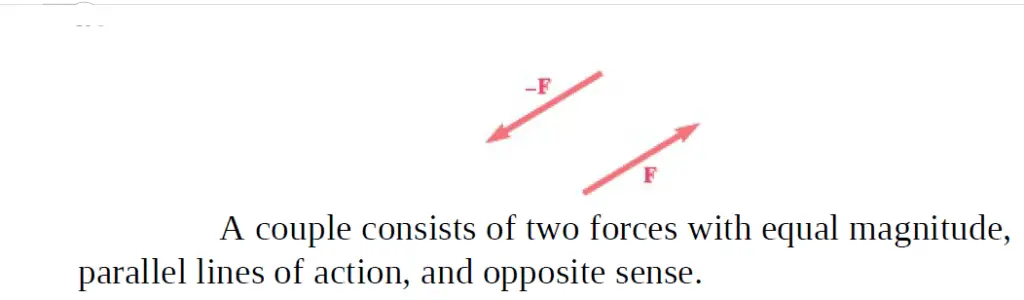# Couple in physics – class 10 notes

Last updated on April 20th, 2023 at 02:25 am

A couple in physics class 10 notes – this is our topic in this post. Now let’s first define a couple in Physics.
Two equal, opposite, parallel forces, not acting along the same line, form a couple. A couple is always needed to produce rotation.figure 1: Two forces F and −F, having the same magnitude, parallel lines of action, and opposite sense, are said to form a couple

Two forces F and −F, having the same magnitude, parallel lines of action, and opposite sense, are said to form a couple (Figure 1). Clearly, the vector sum of the components of the two forces in any direction is zero.

The sum of the moments of the two forces about a given point, however, is not zero. The two forces do not cause the body on which they act to move along a line (translation), but they do tend to make it rotate.

## Couple in physics class 10 – Question & Answer

### Define a couple.

Two equal, opposite, parallel forces, not acting along the same line, form a couple. A couple is always needed to produce rotation.

### Explain how a couple is produced when we open a door

When we open a door, a couple is produced to rotate the door. This couple is produced by the 2 following forces which are equal, opposite, and parallel to each other, but not acting along the same line of action.
1 ) The force we exert at the handle of the door
2) An equal and opposite reaction force produced at the hinge (that is the pivot point)

### Can a single force alone cause the rotation of a pivoted body?

No, that’s not possible. A couple is needed to cause a rotation.

### Examples of Couple in physics

Here are some examples of Couples in physics.

1. couple generated while turning a water tap
2. Turning the key in the hole of a lock
3. winding a watch or clock with a key
4. Turning the steering wheel of a car
5. Pushing the pedals of a bicycle